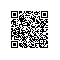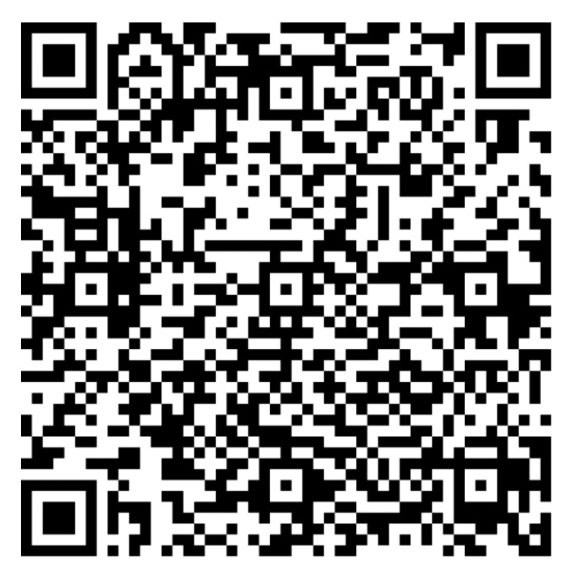# [leetcode/lintcode 题解] 算法面试高频题详解：查找和替换模式

（回想一下，字母的排列是从字母到字母的双射：每个字母映射到另一个字母，没有两个字母映射到同一个字母。）

• 1<=words.length<=50
• 1<=pattern.length=words[i].length<=20

样例1

"mee" 与模式匹配，因为存在排列 {a -> m, b -> e, ...}。
"ccc" 与模式不匹配，因为 {a -> c, b -> c, ...} 不是排列。

样例2

public class Solution {
/**
* @param words: word list
* @param pattern: pattern string
* @return: list of matching words
*/
public List<String> findAndReplacePattern(String[] words, String pattern) {
// Write your code here.
int[] p = F(pattern);
List<String> res = new ArrayList<String>();
for (String w : words)
if (Arrays.equals(F(w), p)) res.add(w);
return res;
}

public int[] F(String w) {
HashMap<Character, Integer> m = new HashMap<>();
int n = w.length();
int[] res = new int[n];
for (int i = 0; i < n; i++) {
m.putIfAbsent(w.charAt(i), m.size());
res[i] = m.get(w.charAt(i));
}
return res;
}
}使用钉钉扫一扫加入圈子
+ 订阅